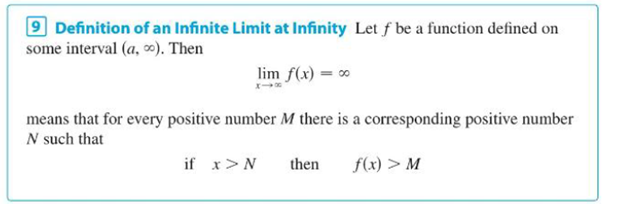Chapter 2.6, Problem 79E

Chapter
Section
Textbook Problem

Use Definition 9 to prove that lim x → ∞ e x = ∞ . Definition 9To determine

To prove: The value of limit of the function limxex=.

Explanation

Definition used:

Let f be a function defined on some interval (a,).

Then limxf(x)= means that for every positive number M there is a corresponding positive number N such that if x>N then f(x)>M.

Graph:

Proof:

Let the function f(x)=ex

Let M=ex1 some x1 is any real number.

M=ex1ln(M)=ln(ex1)ln(M)=x1

Choose N=ln(M) depends on M

Still sussing out bartleby?

Check out a sample textbook solution.

See a sample solution

The Solution to Your Study Problems

Bartleby provides explanations to thousands of textbook problems written by our experts, many with advanced degrees!

Get Started

Find the limit: limx0x+42x.

Calculus: An Applied Approach (MindTap Course List)

In Exercises 4144, determine whether the statement is true or false. 44. 561112

Applied Calculus for the Managerial, Life, and Social Sciences: A Brief Approach

Evaluate the iterated integral: 0101y2(x+y)dxdy

Calculus: Early Transcendental Functions

The percentage formula is written as _______. (6-3)

Contemporary Mathematics for Business & Consumers

Area In Exercises 63-66, find the area of theunbounded shaded region. y=lnx

Calculus: Early Transcendental Functions (MindTap Course List)

In Exercises 110, find the graphical solution to each inequality. x3

Finite Mathematics for the Managerial, Life, and Social Sciences